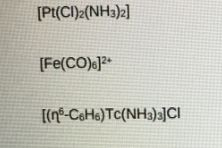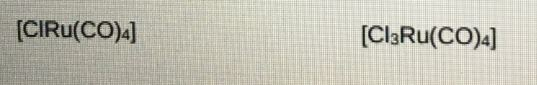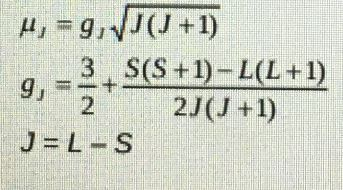# For each of the Following Organometallic Species Calculate the Oxidation State of the Metal Center: Inorganic Chemistry Assignment, CU, UK

 University Coventry University (CU) London Subject Inorganic Chemistry

## Question 1:

• For each of the following organometallic species calculate the oxidation state of the metal center, the total number of valence electrons, and give a diagram that represents the three-dimensional shape.• By considering the extent of back-bonding in the following organometallic carbonyl complexes, which would have the longer C=O bond length. Explain your answer using diagrams and give reference to v CO.• Element-element multiple bonds are less common in heavy element main group compounds. Describe synthetic strategies to overcome this.

## Question 2:

• Explain by lanthanide ions exhibit lines like absorbance and emission spectra in comparison to d-block complexes which have broad spectra.
• What is the valence electron configuration of the Nd (III) ion?
• Calculate the magnetic moment of Nd (111) using the Lande formula below.• Draw a labeled Jablonski diagram showing how a lanthanide excited state is populated.
• Give with examples, two uses of lanthanide complexes, explain your choice of metal and coordination environment.